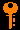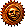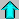# Lecture 7 - Orbital Mechanics III: Energy (10/1/98)Barycenter --- | --- PlanetsReading:

Chapter 2-3 (ZG4)

An orrery, or mechanical model of the solar system.
Key Question:## Investigations:

1. Conservation of Energy
• How is potential energy related to force?
• What is the gravitational potential energy of a particle?
• What is the kinetic energy of a particle?
• How do you compute the total energy of a particle?
• What is the escape velocity from a planet?
• What is the energy equation for a gravitational system?
• What is the difference in energy for elliptical, parabolic, and hyperbolic orbits?

## The Energy Equation:

There are 3 conserved quantities in an isolated mutually gravitating two-body system: linear momentum, angular momentum, and total energy. The energy equation is derived by considering the work done while moving a particle on a path near a gravitating mass (the path integral of F · dr). If we use the substitution

F = m dv/dt

we obtain the expression for the kinetic energy of a body of mass m

K = ½ m v2.

If, on the other hand, we substitute the gravitational force due to a mass M at distance r

F · dr = - G M m / r2

we obtain the expression for the gravitational potential energy

U = - G M m / r.

The total energy is given by the sum (minding the signs)

E = K + U = ½ m v2 - G M m / r

and thus the energy per unit mass is

E/m = (v2 / 2) - (G M / r)

This is the all-important energy equation for a body in orbit at radius r with velocity v around a dominant gravitating mass M.

The limiting case r -> infinity is particularly interesting. Examination of the energy equation shows that the body will come to rest (v=0) at infinity when E/m = 0. Thus, at some finite radius r, if the velocity is equal to the escape velocity

vesc2 = 2 G M / r

then E/m = 0 and the body is marginally bound to mass M, and the orbit is a parabola. If the velocity at r is less than the escape velocity at that r, then the orbit is a closed ellipse. For a velocity greater than the escape velocity, we have an unbound hyperbolic orbit.Prev Lecture ---Next Lecture ---Astr11 Index ---Astr11 Home

smyers@nrao.edu Steven T. Myers